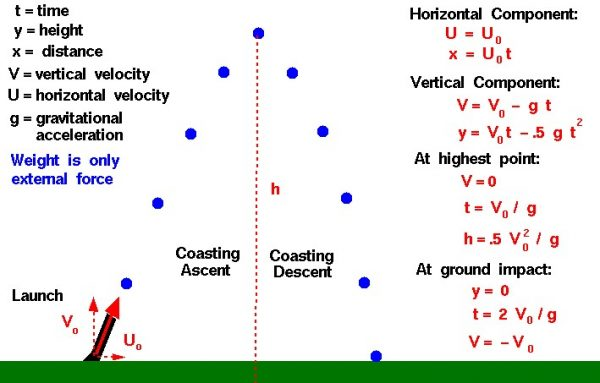# Ballistic Flight EquationsOn Earth a baseball or a soccer ball generates a moderate amount of aerodynamic drag and the flight path is not strictly ballistic. Ballistic flight is, however, a first approximation to the flight of a ball. The actual flight equations including drag are much more complex because the drag is constantly changing throughout the flight. Drag depends on the square of the velocity and the velocity changes during the flight. The drag also depends on the air density which is a function of the weather conditions and altitude.

### Motion

For ballistic flight, the ball is normally inclined at some angle to the vertical (or horizontal) as it is launched. We resolve the initial velocity into a vertical component V0 and a horizontal component U0. The horizontal motion is uniform because there is no external force in the horizontal direction. So, according to Newton’s first law of motion, the horizontal velocity remains a constant and the distance x which the ball travels is given by the velocity times the expended time t:

U = U0

x = U0 * t

In the vertical plane, weight is the only external force acting on the object. Because the weight of the object is a constant, we can use the simple form of Newton’s second law to solve for the vertical motion:

-W = F = m a = m dV/dt

where W is the weight, m is the mass, V is the vertical velocity, t is the time, a is the acceleration, and F is the net external force. The positive direction is upwards, so the weight is preceded by a negative sign. Solving the equation:

dV/dt = – W/m = -g

V = Vo – g t

where g is the gravitational acceleration which is equal to 32.2 ft/sec^2 or 9.8 m/sec^2 on the surface of the Earth. The value of the gravitational acceleration is different on the Moon and Mars. Vo is the initial velocity leaving the launcher. The location at any time is found by integrating the velocity equation:

dy/dt = V = Vo – g t

y = Vo t – .5 g t^2

where y is the vertical coordinate. With this general description of the motion of a ballistic object, we can derive some interesting conclusions.

Notice that the flight equation includes no information about the object’s size, shape, or mass. All objects fly the same in purely ballistic flight. This is similar to Galileo’s principle that all objects fall at the same rate in a vacuum. If drag can be ignored, the flight of the object depends only on the initial velocity and the gravitational acceleration.

### Velocity

At the highest point in the flight, the vertical velocity is zero. From the velocity equation we can determine the time at which this happens:

V = 0

t = Vo / g

The time to maximum altitude varies linearly with the launch velocity. Plugging this time into the altitude equation we obtain:

y = Vo (Vo / g) – .5 g (Vo / g)^2

y = .5 * Vo ^2 / g

The maximum altitude changes as the square of the launch velocity. Doubling the launch velocity produces four times the maximum altitude.